# Understanding an Inverter Simulation

Gold Member
Summary:
I need help understanding how to model an inverter circuit and what to expect given a circuit diagram.
As a step to a solar powered battery backup system I wish to get a better grasp on how DC to AC inverters work. This circuit shown below is a 12V DC to 120V AC inverter. I am doing ltspice simulations first and I am working from a circuit diagram included below I found online here;

https://www.elprocus.com/dc-to-ac-converter-circuit-working-applications/

It is easy to spice this up in ltspice except for the transformer part. I do not understand how to model the center tap and if the transformer is just inductors or if there are other components. Also, I tried to simulate just the 12V DC to 12V AC without the transformer but get nonsensical results.

1) Can I model just 12V DC to 12V AC without the transformer or is the transformer necessary to the circuit?

2) Will this circuit give a sine wave or a modified sine wave?

3) I need help understanding and modeling the center tap transformer part.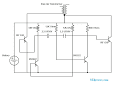I'd like to understand how to get a sine wave with only transistor, caps, diodes, inductors and not using IC's if possible. Thanks for any help or advice!

Baluncore
Summary:: I need help understanding how to model an inverter circuit and what to expect given a circuit diagram.

3) I need help understanding and modeling the center tap transformer part.
The transformer symbol in the diagram has been rotated by 90° which makes it really confusing.

The design of a transformer is described in the LTspice FAQ documentation, but it is probably easier to modify your circuit. If you attach your Ltspice filename.asc file to a post we can edit it and return it, hopefully working. Since it is an ascii text file internally, first change it's extension to filename.asc.txt

In a nutshell, place three inductors; primary Lp1, Lp2 and secondary Lsec. Couple them by placing a K command anywhere on the schematic; Kt Lp1 Lp2 Lsec 1.000 ; Notice they now have dots showing polarity.
Set the inductance to specify the turns ratio. Primary side voltage to secondary voltage here will be about 1 : 10; Because L is proportional to n², (turns squared), give Lsec an inductance of 10² = 100 times the Lp inductance. Transformer current will be too high if L is too low.

•bob012345
Gold Member
Thanks. The entire circuit as drawn is confusing. The drains of the power mosfets go to the transformer but I assume the wire does not intersect the 12V battery but they don't make that clear. Then, it's not clear how or why the center tap connects to the 12V DC. Here is my netlist for ltspice. Thanks for any help.

#### Attachments

• Vibrator2.txt
2.5 KB · Views: 31
Last edited:
Baluncore
Your Vibrator2.txt file is a generic spice file, not an LTspice schematic.asc text file.
It should contain the “wire” commands that draw the schematic.

Attached are example .asc and .plt files that demonstrate a centre tapped transformer.
Remove the .txt extensions to get the LTspice schematic to run and plot.

#### Attachments

• C_tap_0.asc.txt
1.3 KB · Views: 23
• C_tap_0.plt.txt
288 bytes · Views: 23
•bob012345
Baluncore
Here is the cleaned up circuit with the transformer untangled.

I have parameterised most values to make juggling timing and output voltage easier.
The frequency is set by Ct and Rt, (to about 55 Hz).
Given the transformer turns ratio, n=11.0; and the primary inductance, Lp=200mH, Ls=Lp·n² is then computed.

The two diodes protect the transistor base-emitter junctions from negative voltage breakdown, which will occur in the astable for supply voltages over about 5 volts.

A symmetrical circuit simulation may be too well balanced numerically to start cleanly. By specifying an initial condition the simulation starts reliably. The uic flag on the .tran command is needed to use the specified .IC V(A)=5 volts.

Here is the schematic. Attached is the LTspice.asc text file.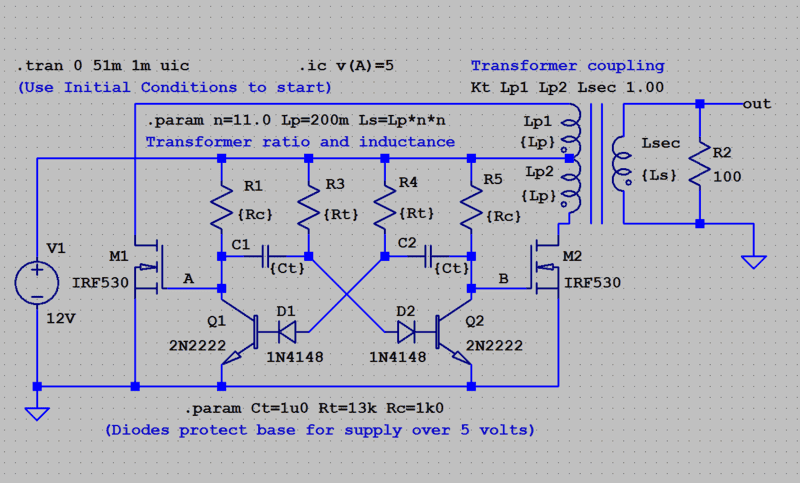#### Attachments

• DC-AC_2.asc.txt
3.3 KB · Views: 21
•jim mcnamara, bob012345, berkeman and 1 other person
Gold Member
Thank you very much! Sorry I sent the txt file. I didn't notice you wanted xxx.asc.txt. I shall run this today.

Gold Member
I ran it and now I understand a few things better. I see that the transformer is integral to the circuit, not just to step up the voltage but to get to AC in the first place and also that this circuit gives a modified sine wave and not a pure sine wave. As a result, it would not work for my solar inverter. Also the center tap hookup makes sense to me now. Next, I'm going to explore pure sine wave inverters. Thanks for the help Baluncore! I appreciate it very much.

•Gold Member
I added a filter to the output and turned the modified sine wave (more like a square wave) into a fairly reasonable sine wave but the current out of the 12V DC source spiked some tenfold. I wonder if its possible to buffer the output from the input so I can change the waveform shape from pulse to sine wave without causing this large current spike? Or, change the waveform shape before it gets to the transformer? Thanks.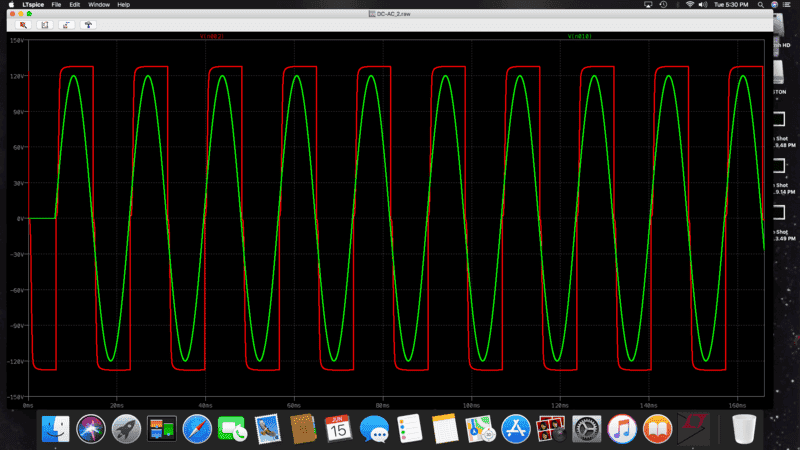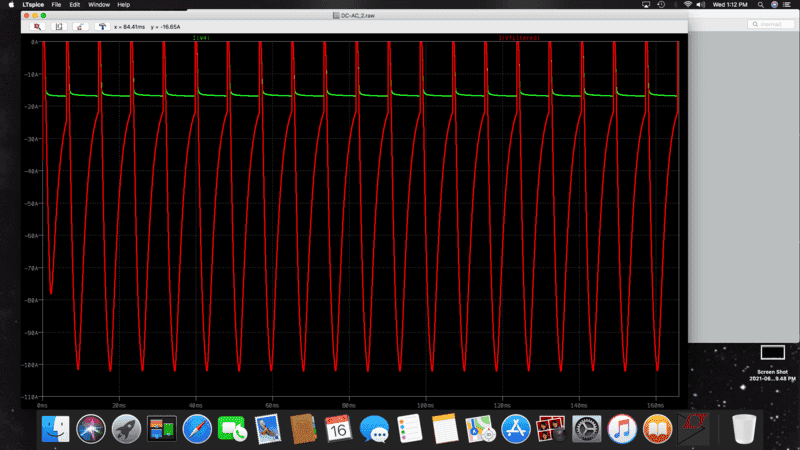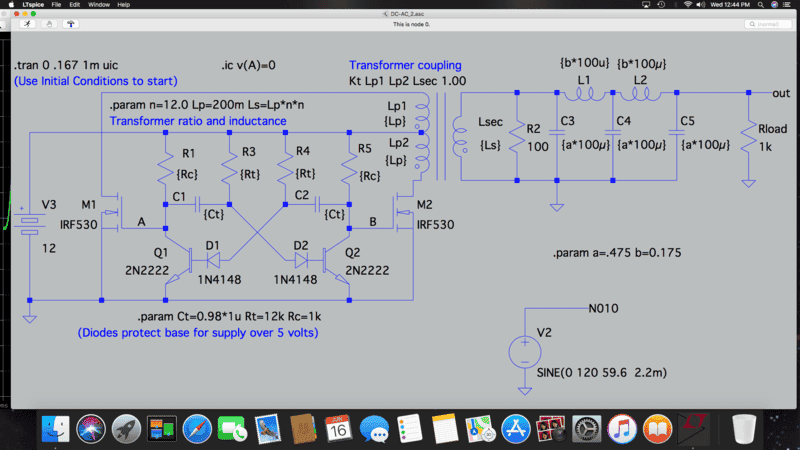DaveE
Gold Member
I added a filter to the output and turned the modified sine wave (more like a square wave) into a fairly reasonable sine wave but the current out of the 12V DC source spiked some tenfold. I wonder if its possible to buffer the output from the input so I can change the waveform shape from pulse to sine wave without causing this large current spike? Or, change the waveform shape before it gets to the transformer? Thanks.View attachment 284557View attachment 284556View attachment 284555
Try removing C3 from your filter.

•bob012345
Gold Member
Thanks, I just tried that but the current still spikes about the same.

Also, I really need to model the 12V source as a battery which in my case is about 10Ah with a maximum
current of around 20A.

DaveE
Gold Member
Thanks, I just tried that but the current still spikes about the same.
OK, then bigger inductor values and smaller capacitor values.

I really need to model the 12V source as a battery which in my case is about 10Ah with a maximum
current of around 20A.
That's not so easy. Battery discharge is very non-linear at the end. Can't you just assume that the battery is charged? In that case a voltage source with a small series resistor is a good approximation. If you want to know what happens when the battery voltage drops, you can just decrease the voltage source value. Otherwise, I think you'll have to integrate the current from the battery to get the amount of charge removed, then apply a non-linear function to that to calculate the source voltage. Expect modelling and convergence problems, and use initial conditions, since there is a sort of feedback implicit here.

•bob012345
Gold Member
OK, then bigger inductor values and smaller capacitor values.

That's not so easy. Battery discharge is very non-linear at the end. Can't you just assume that the battery is charged? In that case a voltage source with a small series resistor is a good approximation. If you want to know what happens when the battery voltage drops, you can just decrease the voltage source value. Otherwise, I think you'll have to integrate the current from the battery to get the amount of charge removed, then apply a non-linear function to that to calculate the source voltage. Expect modelling and convergence problems, and use initial conditions, since there is a sort of feedback implicit here.
Yes, I can assume the battery is charged. I'm interested in steady state. When I put in a small series resistor, the voltage is no longer a fixed 12 volts.

Baluncore
@bob012345
Screen shots are big, hard to read, and can contain hidden circuit values such as parasitic R, L and C.
LTspice has pull down menu 'Tools', select 'Copy bitmap to Clipboard'. For a small readable file paste the bitmap into an image file, crop, and save as .jpg or .png.

Also, attach to your post the schematic.asc file renamed as schematic.asc.txt That way we get the circuit with any hidden values that do not display on the schematic.

There are other inverter topologies that will produce a sinewave, and be less load sensitive.

Gold Member
Thanks. Here is the schematic which I names inverter.asc.txt

#### Attachments

• inverter.asc.txt
4.3 KB · Views: 13
Last edited:
DaveE
Gold Member
When I put in a small series resistor, the voltage is no longer a fixed 12 volts.
Yes, that's exactly what resistors do. What exactly do you want to model about the battery? What is wrong with a fixed DC voltage source?

•bob012345
Baluncore
One problem converting square wave to sine wave with output LC 'T' filters to is that they make an impedance matching network, so the output voltage becomes load dependent. Also, the output filter needs to be selective to the same frequency as the fundamental that was generated by the bi-stable R&C time constant. The sensible way to get the two frequencies to track is to dispense with the bi-stable R&C timing, and take the output of the tuned circuit as feedback to control the power transistors. That is a different topology.

•DaveE, bob012345 and anorlunda
Gold Member
Yes, that's exactly what resistors do. What exactly do you want to model about the battery? What is wrong with a fixed DC voltage source?
I have the battery and want to model the inverter running a 15W AC LED lamp (because those LED bulbs are designed to look like old fashioned lightbulbs and not like flashlights).

Gold Member
One problem converting square wave to sine wave with output LC 'T' filters to is that they make an impedance matching network, so the output voltage becomes load dependent. Also, the output filter needs to be selective to the same frequency as the fundamental that was generated by the bi-stable R&C time constant. The sensible way to get the two frequencies to track is to dispense with the bi-stable R&C timing, and take the output of the tuned circuit as feedback to control the power transistors. That is a different topology.
Understood. Do you think the modified sine wave would run a 15W LED lamp?

Gold Member
Yes, that's exactly what resistors do. What exactly do you want to model about the battery? What is wrong with a fixed DC voltage source?
I mean it oscillates not just gives a reduced DC voltage. That suggests any real battery with some series resistance would ruin the circuit. I also want to model the limited current available from the battery which should be about 20A.

Last edited:
Svein
•bob012345
Baluncore
I have the battery and want to model the inverter running a 15W AC LED lamp
LED lamps designed to run from AC mains convert the AC to DC with a bridge rectifier, storing the charge in a high voltage capacitor. They then use an efficient buck converter to generate a low voltage stable LED current.

The bridge will rectify AC or DC into the capacitor, so you do not need to generate AC or remove the odd harmonics from a square wave to make it sinusoidal. You can feed the output from a square wave oscillator directly to the LED lamp.

You can find or draw the circuit for the LED lamp, then simulate that with AC sine, square and DC inputs.

•bob012345
Gold Member
LED lamps designed to run from AC mains convert the AC to DC with a bridge rectifier, storing the charge in a high voltage capacitor. They then use an efficient buck converter to generate a low voltage stable LED current.

The bridge will rectify AC or DC into the capacitor, so you do not need to generate AC or remove the odd harmonics from a square wave to make it sinusoidal. You can feed the output from a square wave oscillator directly to the LED lamp.

You can find or draw the circuit for the LED lamp, then simulate that with AC sine, square and DC inputs.
Thanks. That's good to know . So I don't need to worry about getting pure AC just to make a simple backup for LED room lamps. For running the TV I think I would though. Yes, I'll try that simulation. Thanks again.

The whole idea for me is to have a few small independent backup power systems to make things appear almost normal in the house during a power outage. For example a few lamps, the TV and Internet and possibly the refrigerator. Secondly, to understand how inverters work.

Last edited:
Gold Member
Thanks. This is a great reference and guide whether I end up building such a system or just buying one. It clarifies the design logic and at the very least I can simulate it for my understanding so thanks again.

Baluncore